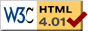### Journée-séminaire de combinatoire

#### (équipe CALIN du LIPN, université Paris-Nord, Villetaneuse)

Le 28 février 2012 à 13h45 en B311, Pavel Salimov nous parlera de : Open problems on maximal pattern complexity of infinite words

Résumé : A pattern T={t_0 < t_1 <...} is a containing 0 finite subset of N. A pattern complexity p_x(T) of an infinite word x: N->{0,1} on pattern T is a number of different words of the form x_{t_0}x_{t_1}... Maximal pattern complexity of x is the function p_x(n) = sup_{|T|=n} p_x(T) of n that takes a maximal value of all pattern complexities of x over patterns of cardinality n. In the talk, I'll describe some properies of this function, compare it to the other well-studied measures of complexity, give an example of calculating it and outline some open problems related to it.

 Dernière modification : Monday 24 January 2022Contact pour cette page : Cyril.Banderier at lipn.univ-paris13.fr